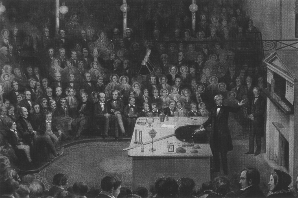# Natural Science - Year I

#### Unit 29: Galileo and MechanicsScience Weblecture for Unit 29

 This Unit's Homework Page History Lecture Science Lecture Lab Parents' Notes

# Science Lecture for Unit 29: Mechanics

### For Class

• Topic area: Physics: Mechanics (motion and force)
• Terms and concepts to know: Force, energy, acceleration, linear force, shear force, torque, contact force, force acting at a distance, equilibrium, relative speed, velocity.
• See historical period(s): Galileo's Mechanics

Lecture Outline

## Experiments with Falling Bodies

1. The definition of terms for motion
1. definition of rate
2. the difference between instantaneous and average rates
3. distance
4. velocity
5. acceleration
2. Divisions of Mechanics
1. Dynamics
2. Statics
3. Kinematics

#### Rates

Before we discuss motion, we need to establish the concept of rate. We use this concept all the time, but the exact measurements of rates of change became a crucial part of our scientific endeavors with Galileo and in contemporaries.

A rate is the amount of change in some quantity that occurs over a given time period. We can measure number of cookies/week consumed by your family; the number of inches of rain per year, and the number of miles per hour your car travels. All of these are examples of rates, and all of them can be plotted on a graph, with the changing quantity on the vertical axis and time running along the horizontal axis.In both cases, the slope of the line gives the rate of change. If the line is straight, we have a linear equation of the form y = mx + b, which you may already have studied in algebra. For the miles per hour graph, we might write this as distance d = vt + d0, where v is velocity, t is time, and d0 is the starting point (on our graph above, d0 = 0, since the starting point is zero). The slope of the line is v, the velocity, and is easy to determine for a straight line.

For the cooky graph, the slope is harder to determine. The line has a different formula, more like number of cookies = -rt2 + r0 (don't worry about how I got the equation for the line; this is an example). A line which has an exponent in its formula is usually curved, which means the slope is changing constantly. We can easily get an average slope between two points on a straight line:

where (x1, y1) and (x2, y2) are any two points on the line.

Let's assume that someone baked 3 dozen cookies for Christmas. You were only allowed to eat them on Christmas Day. The average consumption for the week is 36 cookies/week, but the average consumption for Monday, Tuesday, Wednesday, and Friday was 0 cookies/day, while the rate "spiked" on Christmas, when the consumption rate was 36 cookies per day (or maybe per hour!). Notice that our time periods are getting smaller and smaller. If we want to know what the cooky consumption rate is at a particular instant (say, Christmas Day, exactly one minute after the cookies were made available), it gets trickier. The time period is now 0 length, and dividing by 0 usually is considered an impossible or illegal mathematical operation. Newton saw a way to approximate instantaneous rates, however, by using the mathematical technique he invented (calculus).

Once we understand that velocity is the rate of change in distance, we can talk about a rate of change for velocity itself. When you speed up or slow down, you are changing velocity. This is acceleration, and we can plot it on a graph, too, as velocity (on the y or vertical axis) against time (on the x or horizontal axis).

While the idea of velocity and acceleration may seem simple and obvious to us, actually testing theories on the motion of bodies is quite a challenge. Since both velocity and acceleration are rates with respect to time, we need a good clock which is able to count at least seconds accurately. Unfortunately for Galileo, there was no such clock yet. After he realized that the swing of the pendulum was a fixed time period, however, he was able to use a pendulum to time the balls rolling down the inclined plane.

#### Mechanics

Study Lesson 1: Mechanics at the Learn Physics Today (EasyPhysics) website.  Read only Chapters 2 (Velocity) and 3 (Acceleration) for this unit. We will look at other sections in Mechanics next week.

The science of mechanics is a field within physics. Mechanics includes three major areas:

• dynamics: the study of forces acting on moving bodies
• statics: the study of forces acting on stationary bodies
• kinematics: the study of energy in moving bodies